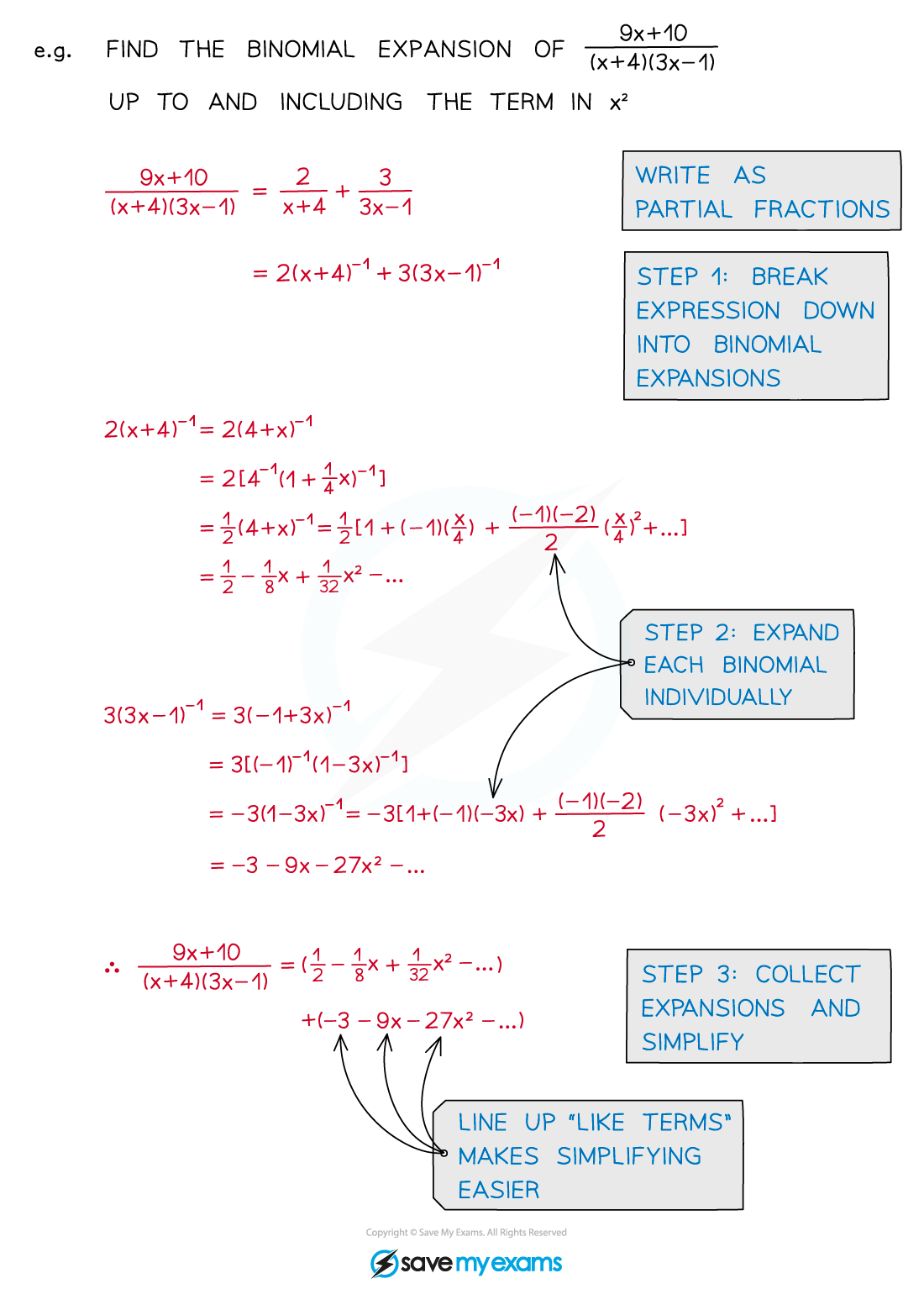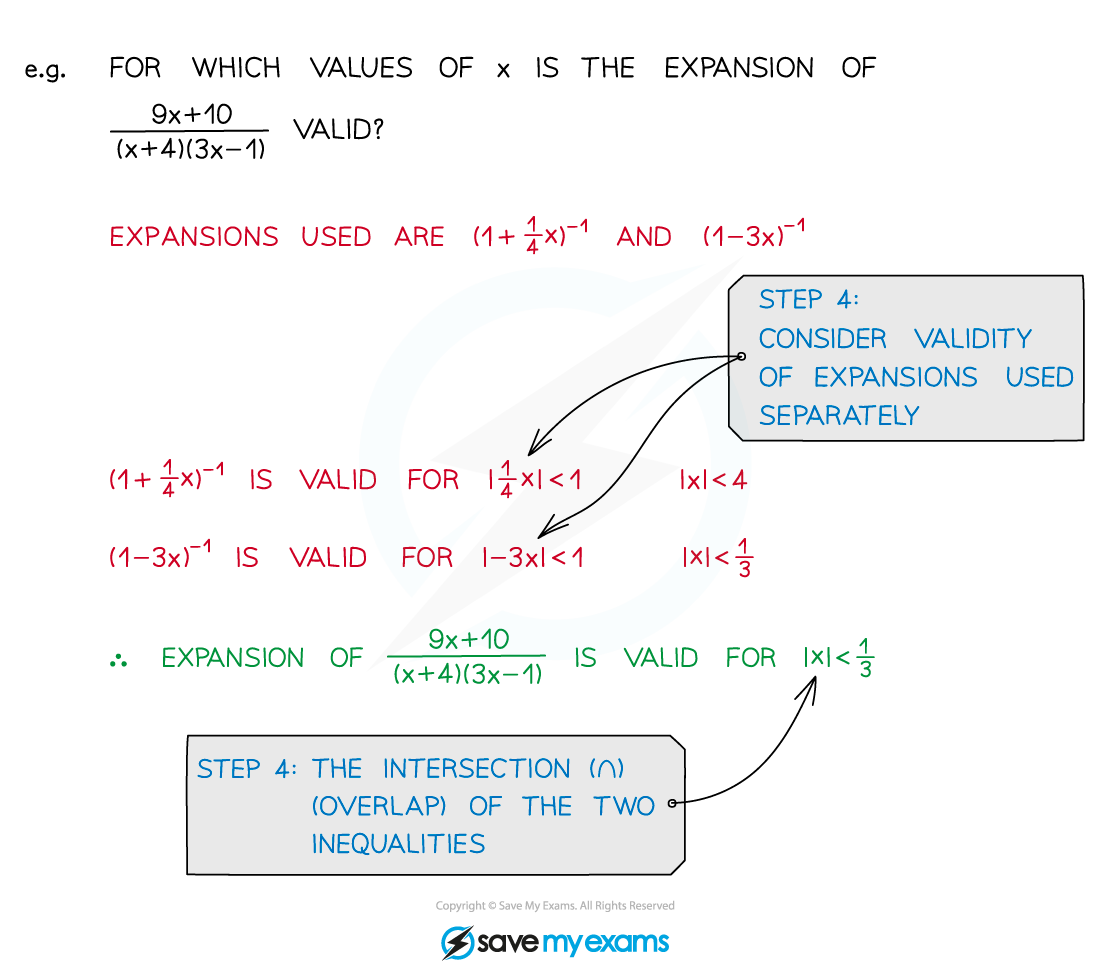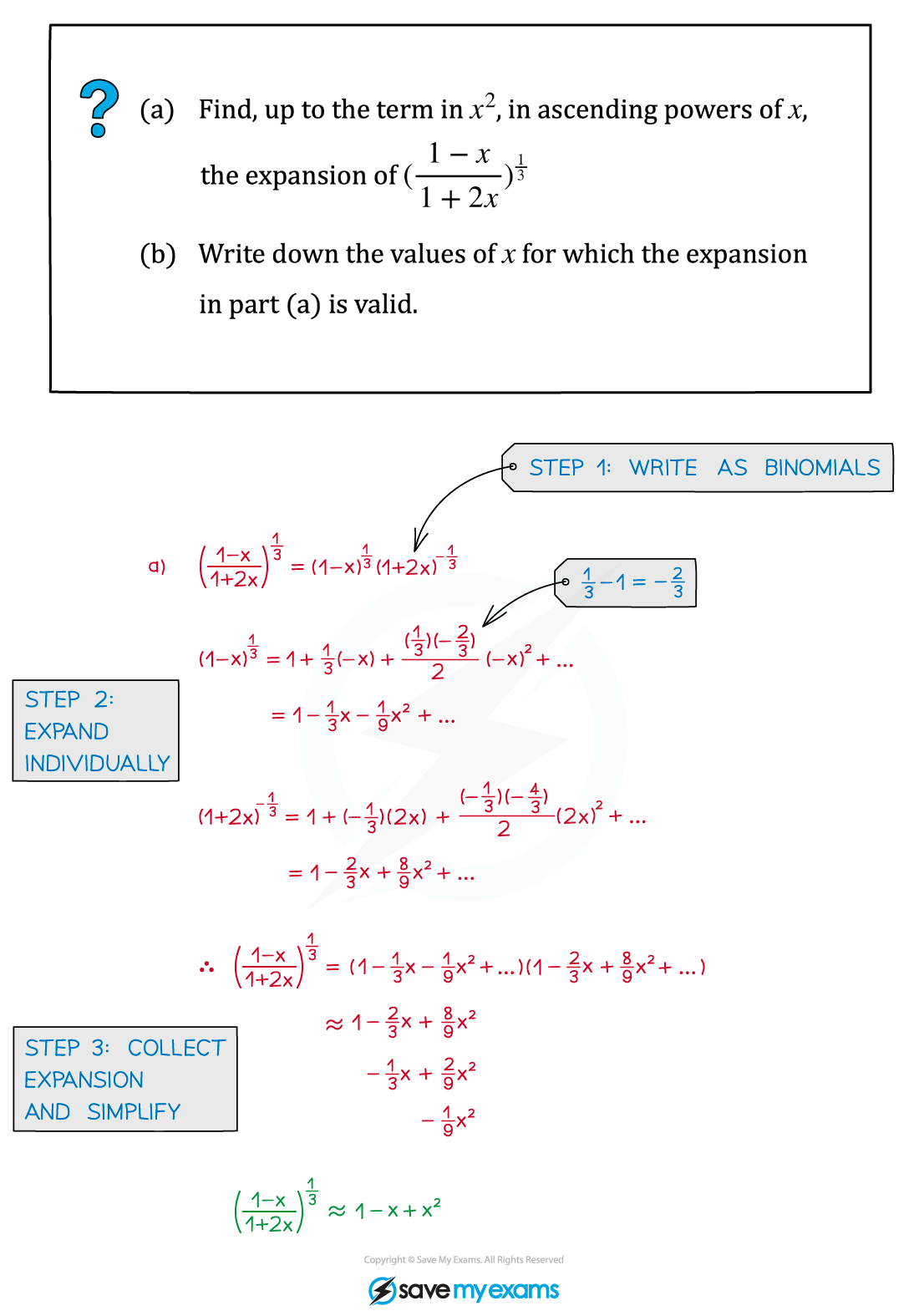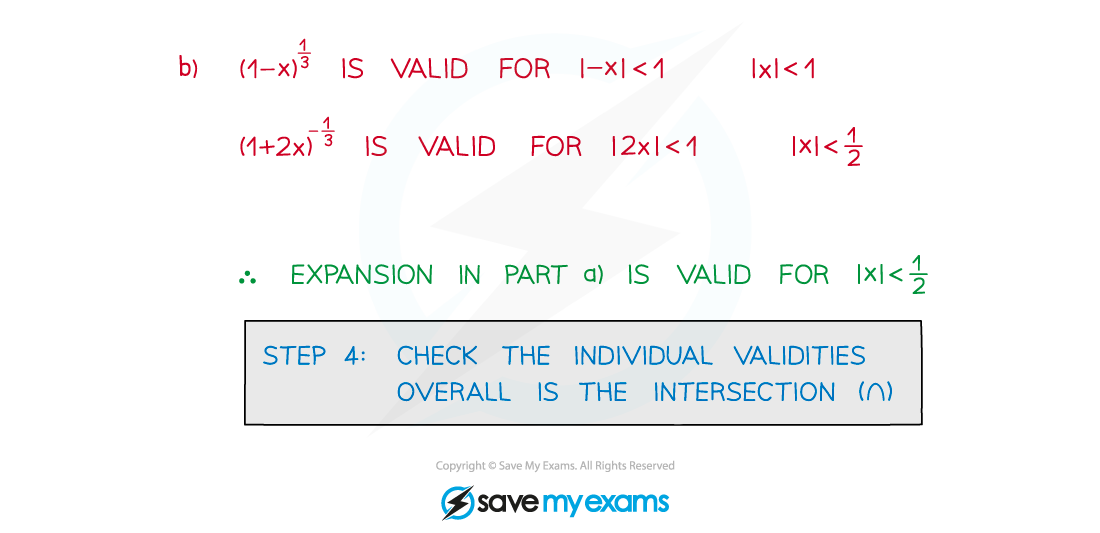# AQA A Level Maths: Pure复习笔记4.2.3 General Binomial Expansion - Multiple

### General Binomial Expansion - Multiple

#### What is the general binomial expansion?

• The general binomial expansion, as given in the formula booklet, is• If n ∈ ℕ then the expansion is finite (see Binomial Expansion)
• Otherwise the expansion is infinitely long
• It is only valid for |x| < 1 (-1 < x < 1)
• Only the first few terms of an expansion are usually needed

#### What is meant by multiple general binomial expansions?

• More than one part of an expression can be a binomial expansion
• These may sometimes be called compound expressions
• The expansion will only be valid for the lowest |x| boundary from all the expansions used

#### How do I use general binomial expansions in complicated expressions?

STEP 1        Break the expression down into binomial expansions

STEP 2        Expand each binomial individually, up to a suitable number of terms

• Be careful with negatives and fractions
• Use brackets as appropriate

STEP 3        Collect the expansions together and simplify

• This could be expanding brackets, collecting like terms, etc
• Ignore any terms of degree higher than required

STEP 4        Check the validity of each binomial expansion

• The overall validity is the intersection (∩)#### How do I work with partial fractions and the general binomial expansion?

• Partial fractions allow rational expressions to be written in a form where the general binomial expansion can then be applied• Validity is an important part of the general binomial expansion#### Worked Example